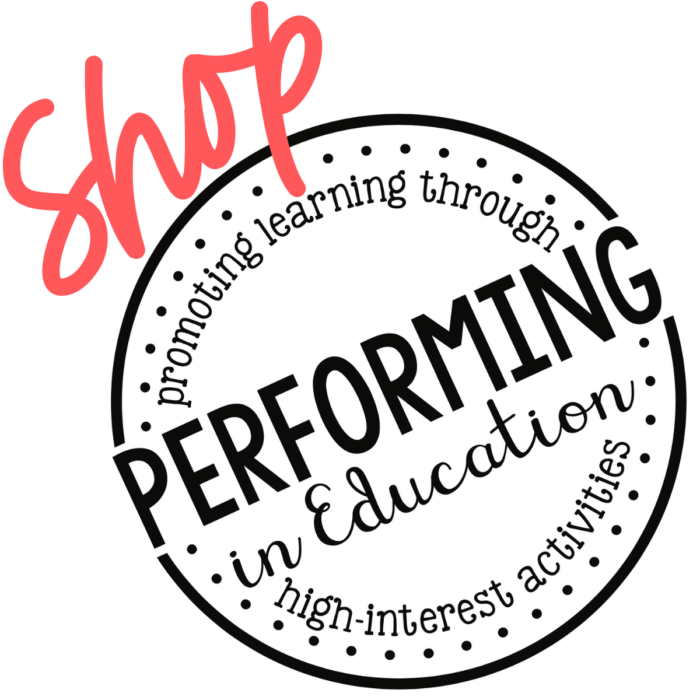# Math Project-Based Learning: Multiplying Fractions & Area | 5th Grade

\$5.00

CCSS Standards: , ,

# Math Project-Based Learning: Multiplying Fractions & Area | 5th Grade

\$5.00

CCSS Standards: , ,

## Description

This 5-day project-based math resource applies math concepts in a real-world scenario to teach students about multiplying fractions and finding area with fractional side lengths. In this 5-day unit, students will use their knowledge of multiplying fractions and mixed numbers to design a theme park. This math project is engaging and authentic. Plus, it’s available as both a digital and print resource.

This project-based math resource is aligned with the following 5th Grade Math Common Core State Standards:

• CCSS.MATH.CONTENT.5.NF.B.4 Apply and extend previous understandings of multiplication to multiply a fraction or whole number by a fraction.
• CCSS.MATH.CONTENT.5.NF.B.4.A Interpret the product (a/b) √ó q as a parts of a partition of q into b equal parts; equivalently, as the result of a sequence of operations a √ó q √∑ b. For example, use a visual fraction model to show (2/3) √ó 4 = 8/3, and create a story context for this equation. Do the same with (2/3) √ó (4/5) = 8/15. (In general, (a/b) √ó (c/d) = (ac)/(bd).
• CCSS.MATH.CONTENT.5.NF.B.4.B Find the area of a rectangle with fractional side lengths by tiling it with unit squares of the appropriate unit fraction side lengths, and show that the area is the same as would be found by multiplying the side lengths. Multiply fractional side lengths to find areas of rectangles, and represent fraction products as rectangular areas.

This resource is available in both Printable & Digital Google Slides versions.

What’s included in this resource:

• Background Knowledge: Using a KWL chart , students will build background knowledge around theme parks.
• Fraction Concepts: Students will review how to multiply a fraction by a whole number.
• Multiplication Models: Students will review visual models of multiplying fractions.
• Multiplying Fractions: Using the included instructional pages, students will learn about multiplying two fractions, a fraction and a mixed number, and two mixed numbers.
• Choose Food Stands: Students will choose two food stands to include in their theme park.
• Determine Recipe Quantities: Using the strategies for multiplying fractions, students will determine the ingredients needed for each recipe being sold at their theme park.
• Finding Area With Tiling and Multiplication: Students will learn how to find area of rectangular shapes with fractional side lengths using tiling and using multiplication.
• Choose Entertainment Options: Students will choose four rides to include in their theme park and calculate the area of each ride.
• Mapping the Theme Park: Students will place all locations on their map and ensure that it is mapped to scale.
• Additional Locations: Students will choose locations to add to their theme park and finalize their park map.
• Wrap Up: Students will write complete a wrap up activity to review their theme park plan.

***************************************************************************

## Standards & Level

CCSS Standards: , ,

## Reviews

There are no reviews yet.

Only logged in customers who have purchased this product may leave a review.

Select